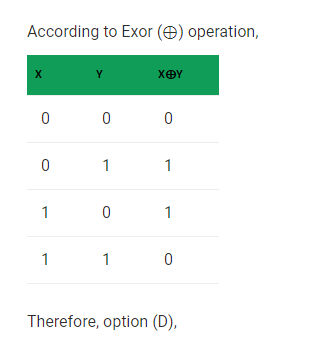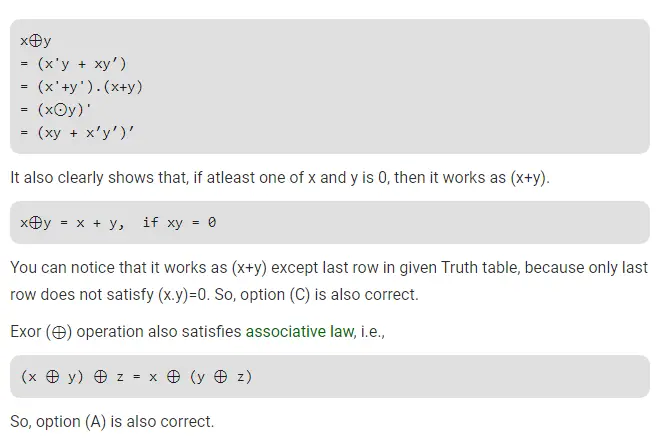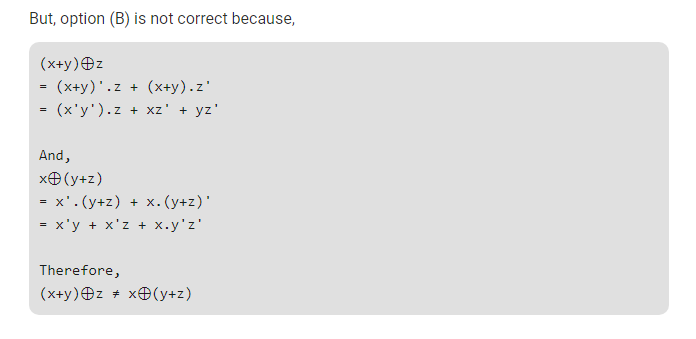# Gate CS-2019 Question Paper With Solutions

Q. 21 Which one of the following is NOT a valid identity?

(A) (x ⊕ y) ⊕ z = x ⊕ (y ⊕ z)

(B) (x + y) ⊕ z = x ⊕ (y + z)

(C) x ⊕ y = x + y, if xy = 0

(D) x ⊕ y = (xy + x′y′)′